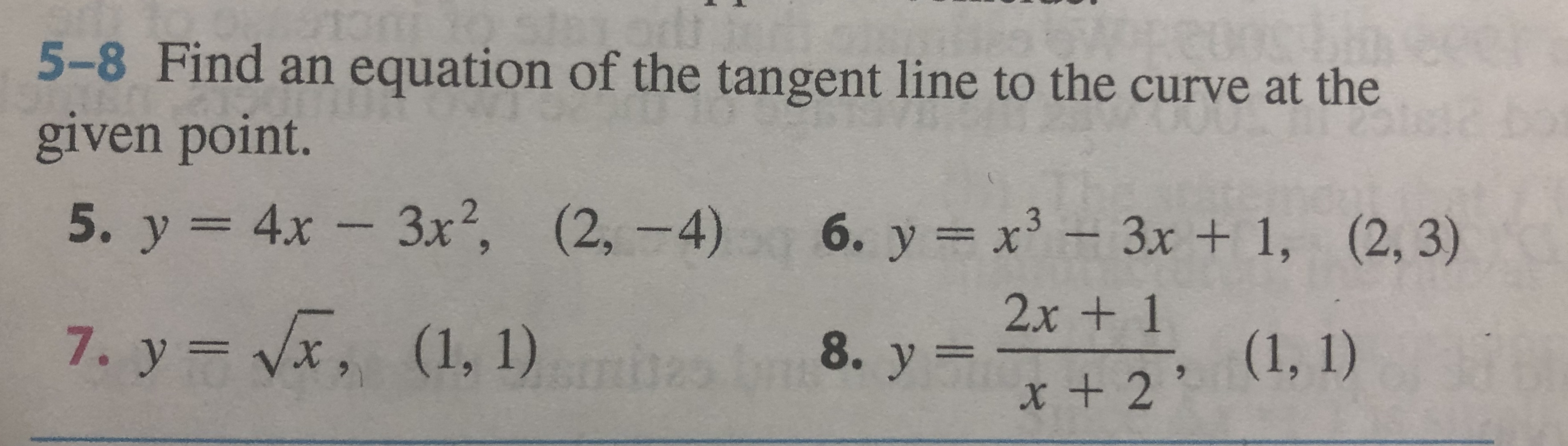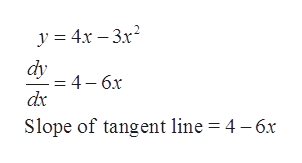# CU5-8 Find an equation of the tangent line to the curve at thegiven point.5. y 4x- 3x, (2, -4)- 3x2,6. y x3-3x + 1, (2, 3)2x + 17. y vx, (1, 1)(1, 1)8. у 3x + 2

Question
3 views

Hi! I need an explanation for number 5 in the photo attached, pleasehelp_outlineImage TranscriptioncloseCU 5-8 Find an equation of the tangent line to the curve at the given point. 5. y 4x- 3x, (2, -4) - 3x2, 6. y x3-3x + 1, (2, 3) 2x + 1 7. y vx, (1, 1) (1, 1) 8. у 3 x + 2 fullscreen
check_circle

Step 1

To find an equation of the tangent line to y = 4x – 3x2 at the point (2, -4).

Step 2

Differentiating y with respect to x which gives the slope of tangent at the point (x, y).help_outlineImage Transcriptionclosey 43x2 dy -= 4-6x dx Slope of tangent line = 4 - 6x fullscreen
Step 3

Let m be the slope of tangent line at point (2, -4). The value of m can be evalua...

### Want to see the full answer?

See Solution

#### Want to see this answer and more?

Solutions are written by subject experts who are available 24/7. Questions are typically answered within 1 hour.*

See Solution
*Response times may vary by subject and question.
Tagged in

### Other